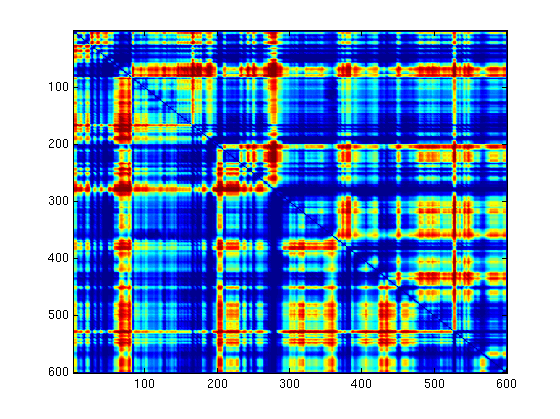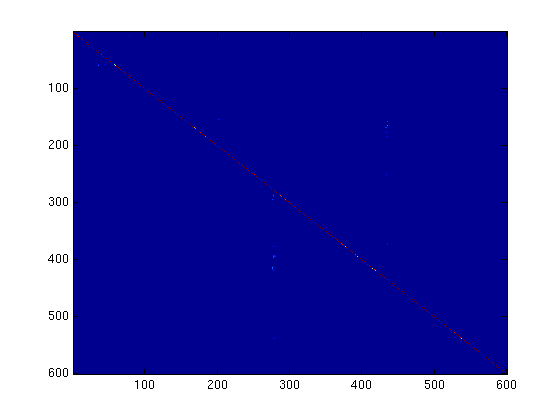# Video Textures

For this project I implemented most of Video Textures by Schold et al.

The core idea of the Video Textures paper is to take in a small amount of similar appearing source footage, do a fair amount of preprocessing, and then have the ability to play arbitrary lengths of visually appealing but stochastically combined video on the fly.

The algorithmic steps required to achieve this are as such:

1. Import your video into MATLAB. It should be a camera-still clip of some object that is likely to periodically return to a visually similar state.
2. Run a n^2 loop to calculate the Sum of Square differences between each frame. That's to say:
• for each frame with each other frame, find the sum of square distances between the values of each pixel.
• Add all of the pixel values for the two frame comparison together. This is the canonical distance between the two frame.
• Store each of the frame distance values for frames x and y at (x,y) in a table we'll call 'distances'.
3. But, this doesn't take into account any temporally surrounding frames. That wouldn't make for good animation with a repetitively moving object. Let's fix it with a neat hack!
We'll convolve it with a diagonal binomial kernal. That will give each value we store a temporal dimension. So in effect any frame comparisons we do now will be of sets of temporally adjacent frames.
Also, congrats on your edge cases!
4. Now we'll have convert that table of distances into a matrix of transitional probabilities.
We'll make a probability table from the distance table using the formula: P(i,j) = exp(-D(i+1,j)/sig)
sig is a bit of a mystery. It can really make the difference between a working video texture and a total failure. The smaller the value the more the formula weights similar transitions over dissimilar transitions.
The value you set is going to have to be higher the fewer good transitions your video contains. I usually set it to around 0.5.
5. You should probably normalize that probability table.
6. You could now also cut out potential transitions which are of less than a certain probability. But normalize again if you do!
7. Now, iterate a through your frames chosing your next one stochastically at every point.

The lighter footage was kind of crappy so these images are not really representative of what the distance etc. matricies looked like. But they're what I have

SSD table for lighter footage:Temporalized version of the above:the final probability transition field:Here are some results. They are varied in quality.

Lighter: original footage bad, but transitions were good

Flag: far too short a source film for far too irregular a subject. Needed tons of sigma and cutoff tweaking to make it do anything. Would probably also require implementation of morphs to look decent.

Me: the source video is a clip of me looking left, then right.